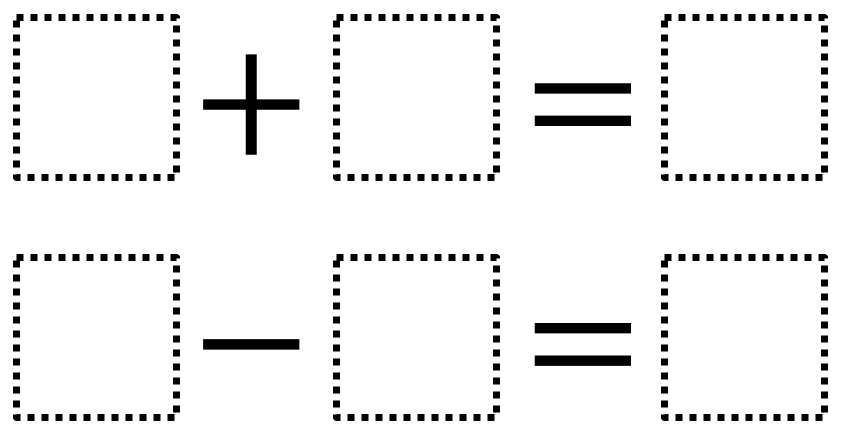Home > Grade 7 > Integer Sums and Differences

# Integer Sums and Differences

Directions: Using the integers -3 to 3, at most one time each, fill in the blanks to make each equation true.### Hint

What do you know about adding and subtracting integers?

### Answer

There are many possibilities. 2 + -3 = -1 and 1 – 3 = -2; 1 + 2 = 3 and -3 – (-1) = -2

Source: Jeanmarie Mullen

## Converting a Fraction to a Decimal

Directions: Using the digits 1 to 9, at most one time each, place a digit …

### 3 comments

1.The second solution is incorrect. -3 – 1 = -4
It should read -3 – (-1) = -2

•Thanks for pointing this out Kara. I have edited the solution.

2.wrong answer